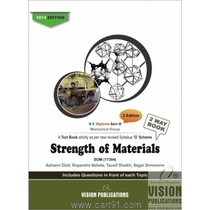# Strength Of Materials

(Inclusive of all Taxes)

Rs. 315

## Details

• Language English

• ISBN 13 9789350161920

• Edition 2

• Publish Year 2014

• Book Format Printed

• Publishers Vision Publication

1. Mechanical Properties of Materials, Simple Stresses and Strains
1. Introduction
2. Mechanical Properties of Materials2
2.2 Modulus of Elasticity
3. Bars of Composite Sections
3.1 Shear Stress in Rivets
3.2 Behaviour of Ductile Material under Load
3.3 Behaviour of Brittle Material under Load
3.4 Some Important Concepts /Terms
Numericals on Stress-Strain Curve
3.5 Concept of Linear Strain, Lateral Strain and Poisson’s Ratio
3.9 Elastic Constants
Numericals on Elastic Constants
3.10 Temperature Stresses and Strain
Numericals on Thermal stresses
3.11 Thermal Stresses in Composite Bar
3.12 Hoop-Stress and Strain
4. Buckling
4.1 Important Definitions
4.2 Equivalent Length of the Column79
4.3 Classification of Columns
4.4 Euler’s Theory for Long Column
4.5 Rankine’s Formula
Solved Example
2. Principal Stresses and Planes
1. Principal Planes
2. Principal Stress
2.1 Stresses on an Oblique Section of a Body Subjected to a Direct                Stresses on One Plane
2.2 Stresses on an Oblique Section of a Body Subjected to Direct Stresses       in Two Mutually Perpendicular Direction
2.3 Stresses on an Oblique Section of a Body Subjected to Direct and             Shear Stress on One Plane
2.4 Stresses on an Oblique Section of a Body Subjected to Direct and             Shear Stress on Mutually Perpendicular Plane
2.5 Stresses on an Oblique Section of a Body Subjected to a Simple               Shear
2.6 Mohr’s Circle Method for Finding Principle Stresses and Planes
Solved Problems
3. Thin Cylinder
3.1 Failure Pattern of Thin Cylindrical Shell
3.2 Stresses in a Thin Cylindrical Shell
3.4 Strains in the Thin Cylindrical Shell
Solved Examples
5. Bending Moment and Shear Force
1. Introduction
1.2 Shear Force (SF)
1.3 Bending Moment (BM)
2. Shear Force Diagram (SFD) and Bending Moment Diagram (BMD)
3. Reaction Calculation
3.1 Reaction Calculation for Simply Supported Beam
Solved Examples
3.2 Reaction Calculation for Cantilever
3.3 Reaction Calculation for Over Hanging Beam
Solved Examples
Problems to find point of Contraflexure
4. Moment of Inertia
1. Introduction
2. Polar Moment of Inertia (IZZ or Ip)
4. Theorem of Moment of Inertia
5. M.I. of Solid and Hollow Rectangular Section
Solved Examples
6. M.I. of Solid and Hollow Circular Section
Solved Examples
7. M.I. of Semi-Circular Section
Solved Examples
8. M.I. of Quarter Circle
Solved Examples
9. M.I. of Triangular Section
Solved Examples
10. Moment of Inertia of Composite Figures/Plane Laminas
11. Formulae of Centroid
12. Formulae of M.I
Solved Examples
5. Bending Stresses
1. Introduction
2. Theory of Simple (Pure) Bending
2.1 Stress Distribution Diagrams for Cantilever and Simply Supported Beams
2.2 Assumptions in the Theory of Bending
2.3 Flexural Formula or Equation of Bending
2.4 Moment of Resistance
2.5 Section Modulus (Z)
3. Concept of Direct and Transverse (Vertical) Shear Stress
3.1 Direct Shear Stress
3.2 Transverse (vertical) Shear
3.3 Shear Stress Equation
3.4 Shear Stress Distribution for a Rectangular Section
3.5 Shear Stress Distribution for a Circular Section
3.6 Shear Stress Distribution for Hollow Rectangular Section
Solved Examples
6. Direct and Bending Stresses
1. Introduction
1.3 Direct Stresses
1.4 Bending Stresses
1.5 Maximum and Minimum stresses (Resultant stresses)
2. Stress Distribution Diagram
3. Condition for no Tension in the Section
Solved Examples
7. Torsion
1. Introduction
2. Concept of Pure Torsion
3. Assumptions in Theory of Pure Torsion
4. Torsional Equation for Solid Circular Shaft
5. Power Transmitted by Rotating Shaft
6. Parameters/Comparison of Solid and Hollow Shaft
Solved Examples
solved paper of winter 2013

• Phase Diploma

• Stream Engineering

• Branch Mechanical

• Standard/Year Secondyear

• Semester Iii

• Medium English

• Board/University Pune

• Subject Strength Of Materials

#### Reviews

• No Reviews written for this product. Be the first to write a review.